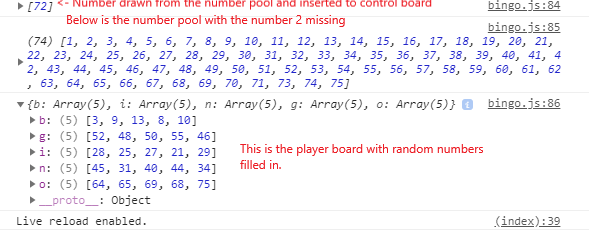Mikke.Learns

# Coding project: Bingo. Part 2

April 28th, 2019

I stared at the type: entry-hyperlink id: c7AgEpet6sD3x1RAj6jl7W9, trying to figure out how to make this work with code.

I felt I had a pretty good plan for the initial setup, but had no idea how to make the actual “look for 5 in a row” function to work. I hoped the answer would come eventually, and started coding.

My first mistake was to start with an array, containing all the numbers to be drawn.

```/* This gets to messy and takes to long... let bingoNumbers = [ 1, 2, 3, 4, 5, 6, 7, 8, 9... ] */```

That would take a long time. So I switched it out for a while loop. To make it work I first had to create the appropriate variables.

```// The amount of number pieces let numberPieces = 75; // The number pool to draw from let numbersToDraw = [] // The control board let controlBoard = []```

One variable for the total number of pieces (numberPieces). One variable for all the number pieces (numbersToDraw (1-75)) and one variable for the control board.

The idea is that when the player presses “draw”, a random number will be moved from the numbersToDraw variable to the controlBoard variable.

A while loop helps us fill the numbersToDraw array based on the numberPieces variable.

```// Fill the numbersToDraw array with numbers while (numberPieces > 0) { numbersToDraw.unshift(numberPieces); numberPieces--; }```

#### The draw function

Next up was creating the function that handles the drawing mentioned above.

I created a function that takes no arguments. First, it creates a random number based on the array length of numbersToDraw (which will decrease for each draw). Then it uses this random number to splice a value from numbersToDraw and pushes it to the controlBoard variable.

```// Draw a random number from numbersToDraw const drawNumber = function () { let randomNumber = Math.floor((Math.random() * numbersToDraw.length) + 1); // Why do this push an array, and not just the number? controllBoard.push(numbersToDraw.splice(randomNumber, 1)); }```

At first, I could not get this to work properly. Can you see why?

When using the following code, I was given an array with the value inside, and not the value itself.

`controllBoard.push(numbersToDraw.splice(randomNumber, 1));`

The simple reason is that the splice method returns an array. So all I had to do, was to add a zero in brackets at the end. This indicates that we want the value at position zero (first) in the array and not the array itself.

```const drawNumber = function () { let randomNumber = Math.floor((Math.random() * numbersToDraw.length) + 1); // This works. Why? It is still an array... so we need to get the first item in the array... controllBoard.push(numbersToDraw.splice(randomNumber, 1)); }```

#### The player board

Now, this is a challenge. I have no idea if this is a proper way to do it, or a good one, but I have to start somewhere.

My first thought was to create an object, since they are representations of real-life objects like a car, a house, a person or, in this case, a player board. I made one array for each letter.

```// The player board let playerBoard = { b: [], i: [], n: [], g: [], o: [] }```

Now came the biggest challenge yet. Filling the player board with random numbers is easy enough, but I had to make sure that there were no duplicates. And besides, each letter on the board represents numbers within a specific range. B is 1 - 15, I is 16 - 30 and so on.

I spent a good amount of time on this one, creating a couple of infinite loops and messing up the code.

I found out that I needed an additional function for giving me a random number within a range. The number 15 is the total numbers in the game (75) divided by the letters (bingo(5)). The function takes one argument, which is the number to start from.

```// Create a random number to use in filling the player board const randomNumber = function (startNumber) { return Math.floor((Math.random() * 15) + startNumber); }```

I then started working on the function for filling the player board. The challenge here was to make sure that there were no duplicate numbers. I solved that with an extra while loop and an if else statement.

```// A function to fill a row in the player board const fillPlayerBoard = function (array, number) { randomNum = randomNumber(number); counter = 0; while (counter < 5) { if (array.indexOf(randomNum) == -1) { array.push(randomNum); counter++ } else { randomNum = randomNumber(number); } } }```

In this function, I first use my randomNumber function to create a random number.

I use this random number in if/else statement inside the while loop, to check if this number already exists in the array being filled. If it’s there, the if statement is false and the else statement creates a new random number for us to use.

If the random number is not in the array, we insert it and at the same time increase our counter.

When the counter reaches 5, we have filled the array passed into the fillPlayerBoard function with five unique random numbers within a range and the while loop stops.

I use this function to fill all the arrays in the playerBoard object with numbers in the corresponding range.

```fillPlayerBoard(playerBoard.b, 1); fillPlayerBoard(playerBoard.i, 16); fillPlayerBoard(playerBoard.n, 31); fillPlayerBoard(playerBoard.g, 46); fillPlayerBoard(playerBoard.o, 61);```

I am sure I can make this function better in the future, but right now I am happy. Everything seems to be working as intended.

Now comes the difficult part. How to check the player board against the number that is drawn, and how to check for five in a row horizontally, vertically and diagonally. And there is the issue of the middle piece being “free”.

Here we can see that everything seems to be working in the console: